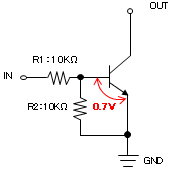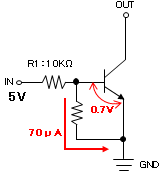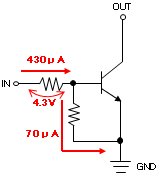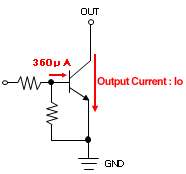FAQ's
 How do you calculate the Base current of a digital transistor?  Using ROHM’s digital transistor DTC114EKA as an example:As forward current flows through E-B, approximately 0.7V exists (at 25C) between E and B. Since the internal resistor R2 is connected in parallel, the voltage across is identical. Therefore, the current flowing through R2 is calculated as follows:IR2 = 0.7V / 10kΩ = 70µAWhen 5V is supplied to the Base at IN a voltage of 4.3V (5V-0.7V) exists across R1, resulting in a current of:IR1=4.3V / 10KΩ = 430µATherefore, 430µA-70µA=360µA flows through the Base of the transistor.For stable operation the input voltage Vin must be adjusted in order to maintain an output current 10-20 times the Base current or lower. If sufficient output current cannot be obtained a digital transistor with lower R1 should be used.At 25ºC the forward voltage (VF) across E-B is approximately 0.7V. However, please note that VF will decrease by about 2.2mV for each degree 1ºC above 25ºC. For example, at an ambient temperature of +50ºC the forward voltage is approximately: 0.7V-(50ºC-25ºC)×2.2mV=0.645V. Therefore, it is imperative to consider the effects of ambient temperature on VF. Please note that there is a voltage tolerance of ±0.1V.In the case of internal resistors R1 and R2 a tolerance of ±30% exists. Please calculate accordingly. Products: Digital Transistors